ChemistryShowing results 41 to 50 of 52, on page 5 of 6 Atomic Structure Electrochemistry Equilibrium Gases, Liquids, and Solutions Thermochemistry
 DESCRIPTION EQUATION Radii of stable orbits in the Bohr modelReaction Quotient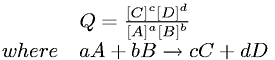Relationship between Energy and Principal Quantum Number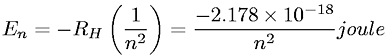Relationship between Equilibrium Constant and Cell Voltage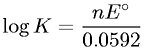Root Mean Square Velocity of Gas Molecules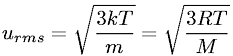Rydberg EquationSpecific Heat Capacity to Heat EquationSpeed of Light to Wavelength and Frequency RelationshipTemperature in Kelvin from Degrees Celsius Conversion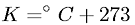Total Gas Pressure as Sum of Partial PressuresGo to page: 1  2  3  4  5  6